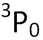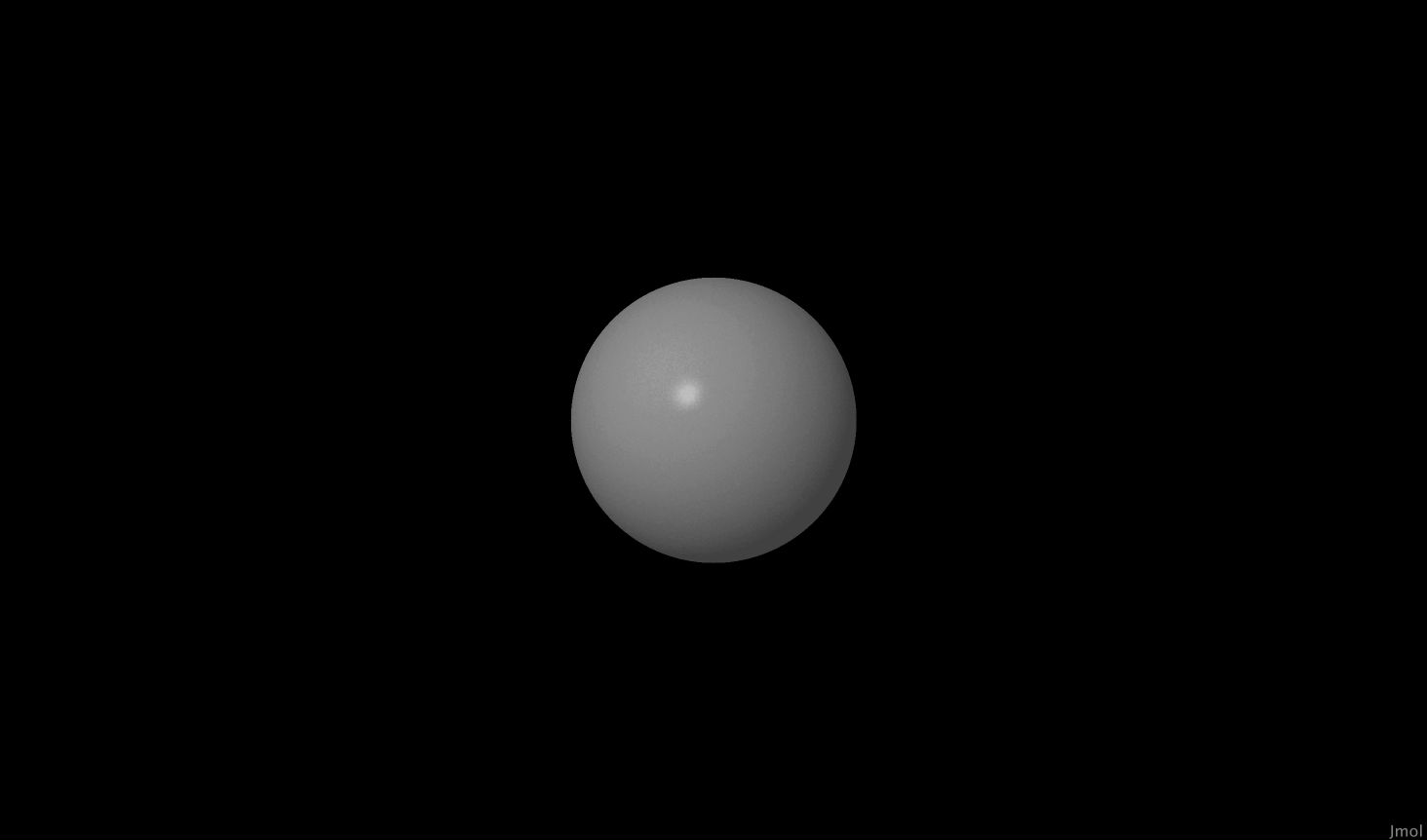# C

Click on the   icon info to get details.

Species data
 Common Formula C Stoichiometric Formula C Name Carbon atom Mass 12.00000 a.m.u Charge 0 CAS 7440-44-0 Inchi InChI=1S/C InchiKey OKTJSMMVPCPJKN-UHFFFAOYSA-N State Ground StateISM Abundance
log10 Abundance Reference Source Name Source Type Link
Polarizability
 Definition: total Value (Å3): 1.67 ±0.03 Method: Reviews and Evaluations Origin: Bibliography Reference: Schwerdtfeger, Peter et al. ;2019;Molecular Physics;117, 1200-1225 Definition: total Value (Å3): 1.76 ±0.0352 Method: Calculations Origin: Bibliography Reference: Lide, D. R.. ;2001;
Dipole moment
 Value (D): 0 Method: N/A Origin: Database : NIST COMPUTATIONAL CHEMISTRY COMPARISON AND BENCHMARK DATABASE Reference:
Enthalpy of formation
 T (K): 0 Value (kJ.mol-1) : 711.198 ±0.45 Method: Reviews and Evaluations Origin: Other database Reference: T (K): 298 Value (kJ.mol-1) : 716.67 ±0.45 Method: Reviews and Evaluations Origin: Other database Reference: T (K): 298 Value (kJ.mol-1) : 716.68 ±0.45 Method: Measurements Origin: Other database Reference: T (K): 0 Value (kJ.mol-1) : 711.19 ±0.45 Method: Measurements Origin: Other database Reference:
Desorption energy
 Emean (K): 10000 ±3000 E min (K): 0 E max (K): 0 Pre-exponential factor (s-1): 0.00E+0 Method: Calculations Origin: Bibliography Reference: Wakelam, V. et al. ;2017;ArXiv e-prints;, Type of surface: H2O Description: To estimate the unknown binding energies (for most of the radicals for example), we have developed a model founded on the stabilization energy of the complex between the various species and one water molecule. Then, we assume that the binding energy of the species with ASW is proportional to the energy of interaction between this species and one water molecule. To determine the proportionality coefficients, we fit the dependency of the experimental binding energies versus the calculated energies of the complexes for 16 stable molecules. Uncertainties in ED is estimated to be 30%. The preexponential factor is to be computed using the Hasegawa et al. (1992) approximation. Evaluation: Emean (K): 15981 E min (K): E max (K): Pre-exponential factor (s-1): 7.38E+14 Method: Reviews and Evaluations Origin: Bibliography Reference: Minissale, Marco et al. ;2022;ACS Earth and Space Chemistry;, Type of surface: Compact Amorphous Solid Water Description: Uncertainties are discussed in Minissale et al. (2022) Evaluation: Emean (K): 15981 E min (K): E max (K): Pre-exponential factor (s-1): 7.38E+14 Method: Reviews and Evaluations Origin: Bibliography Reference: Minissale, Marco et al. ;2022;ACS Earth and Space Chemistry;, Type of surface: SiO2 Description: Uncertainties are discussed in Minissale et al. (2022) Evaluation: Emean (K): 800 E min (K): 0 E max (K): 0 Pre-exponential factor (s-1): 0.00E+0 Method: Estimation Origin: Bibliography Reference: Tielens, A.G.G.M. et al. ;1982;Astronomy and Astrophysics;114, 245-260 Type of surface: H2O Description: This binding energy was listed in the original OSU gas-grain code from Eric Herbst group in 2006. The pre-exponential factor is not given. It can be computed using the formula given in Hasegawa et al. (1992). Evaluation: Emean (K): 4000 E min (K): 0 E max (K): 0 Pre-exponential factor (s-1): 0.00E+0 Method: Estimation Origin: Bibliography Reference: Ruaud, M. et al. ;2015;Monthly Notices of The Royal Astronomical Society;447,4004-4017 Type of surface: H2O Description: The value by Tielens & Hagen (1982) of 800 K is very likely underestimated. The pre-exponential factor is not given. It can be computed using the formula given in Hasegawa et al. (1992). Evaluation:
Diffusion energy
 E (K): 1020 ±40 Pre-exponential factor (cm2 s-1): 0.00E+0 Method: Measurements Origin: Bibliography Reference: Tsuge, Masashi et al. ;2023;Nature Astronomy;, Substrate: Compact ASW Type of diffusion: Surface Description: The diffusion energy is valid for physisorbed C atoms Evaluation: